# A hammer

A hammer weighing 500 g hits the nail at a speed of 3 m/s. What is the average force acting on the nail, which penetrates the board to a depth of 5 cm?

F =  45 N

### Step-by-step explanation:Did you find an error or inaccuracy? Feel free to write us. Thank you!Tips to related online calculators

## Related math problems and questions:

• A steel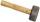A steel hammer with a weight of 0.5 kg hits the nail at a speed of 3 m/s. How deep the nail penetrates the wood if the hammer acts on the nail with an average force of 50N.
• Shot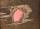Shot with a mass 43 g flying at 256 m/s penetrates into the wood to a depth 25 cm. What is the average force of resistance of wood?
• The shooter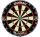The shooter heard the impact of the bullet on the target in one second after the shot. The bullet was moving at an average speed of 500 m/s. Calculate with speed of sound of 340 m/s. Determine the distance of the target.
• Harmonic oscillator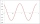Calculate the total energy of a body performing a harmonic oscillating motion if its mass is 200 g, the amplitude of the deflection is 2 cm and the frequency is 5 Hz. Round the result to 3 decimal places.
• CarAt what horizontal distance reaches the car weight m = 753 kg speed v = 74 km/h when the car engine develops a tensile force F = 3061 N. (Neglect resistance of the environment.)
• Braking distance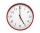The car travels at an average speed of 12 km/h and detects an obstacle 10 m in front of it. At 1 m in front of the obstacle it already runs 2 km/h. What is the braking distance? What is the required deceleration for stop in: A) 1m B) 1s?
• Two airportsTwo airports are 2690 km away. From the first airport, the airplane flies at a speed of 600 km/h, from a second airplane at a speed of 780 km/h. When will they meet if they flew at 10:00? How far from the first airport?
• Two citiesThe car goes from city A to city B at an average speed of 70 km/h, back at an average speed of 50 km/h. If it goes to B and back at an average speed of 60 km/h, the whole ride would take 8 minutes less. What is the distance between cities A and B?
• Free fall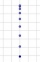The elevator car weighing 720 kg is torn off at a height of 125 m and begins to fall in a free fall. How much kinetic energy does the elevator car have when it hits the ground floor of the building, when the free fall of the car lasted 5 s?
• Two trainsThrough the bridge, long l = 240m, the train passes through the constant speed at time t1 = 21s. A train running along the traffic lights at the edge of the bridge passes the same speed at t2 = 9s. a) What speed v did the train go? b) How long did it take
• A carA car weighing 1.05 tonnes driving at the maximum allowed speed in the village (50 km/h) hit a solid concrete bulkhead. Calculate height would have to fall on the concrete surface to make the impact intensity the same as in the first case!
• Athletic competitionIn a 400 meter athletic competition, a participant covers the distance as given below. find the average speed? first 80 meters 10 m/s next 240 meters 7.5 m/s last 80 meters 10 m/s
• Average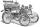What is the average speed of the car, where half of the distance covered passed at speed 66 km/h and the other half at 86 km/h.What overload in g (g-force) has passed the pilot if he accelerated from 0 to 600 km/h in 3 seconds?The driver of a car is to get to 608 km distant city. From atlas found that 162 km will have to pass through the cities at average speed 48 km/h. Remainder of the journey pass outside the cities at average speed 116 km/h. Calculate how many hours it willWhat is the hydrostatic force applied to an area of 30 cm2 in the water at a depth of 20 m? (Water density is 1000 kg/m3)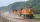The train speed is decreased during 50 sec from 72 km/h to 36 km/h. Assuming that the train movement is equally slowing, find the the acceleration and the distance that it travels at.